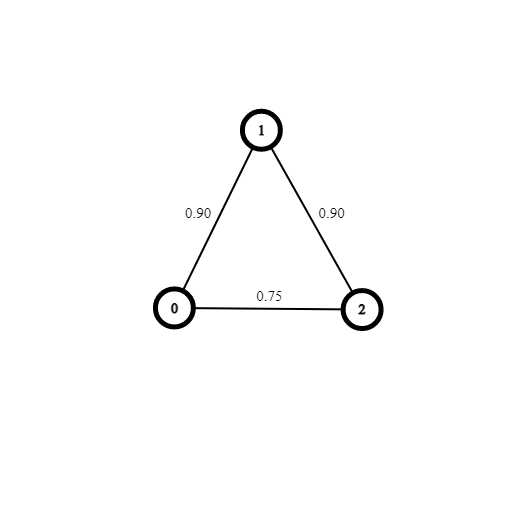New update is available. Click here to update.Close
Topic list
Path With Maximum Probability
MEDIUM
25 mins2 upvotes
Graph
Topics (Covered in this problem)
Problem solved
Skill meterGraph
-
-
Other topics
Problem solved
Skill meterStrings
-
-Matrices (2D Arrays)
-
--
-Sorting
-
-Binary Search
-
-Stacks & Queues
-
-Trees
-
-Dynamic Programming
-
-Greedy
-
-Tries
-
-Arrays
-
-SQL
-
-Binary Search Trees
-
-Heap
-
-Bit Manipulation
-
-
Solve problems & track your progress
Checkout your overall progress in every topic here
BecomeSensei
in DSA topics
Open the topic and solve more problems associated with it to improve your skills
Check out the skill meter for every topic
See how many problems you are left with to solve for cracking any stage. Score more than zero to get your progress counted.

# Path With Maximum Probability

Contributed by
Vivek Kumar Mehta
Medium0/80
Avg time to solve 25 mins
Success Rate 75 %Share2 upvotes

## Problem Statement

#### You are given an undirected unweighted graph and an array 'sProability' which denotes the probability of traversing edges such that 'sProability[i]' denotes the probability of traversing ith edge. You are given the start and end vertex, You need to determine the maximum path probability on going from start to end vertex if there is no path from start to end return 0.

Detailed explanation ( Input/output format, Notes, Constraints, Images )##### Sample Input 1
``````1
3 3 0 2
0 1
1 2
0 2
0.9 0.9 0.75
``````
##### Sample Output 1
``````0.810000
``````
##### Explanation for Sample Output 1:
``````For the test case 1:

The graph is as follows:
````````````Path with maximum probability from 0 to 1 is 0->2->1. Hence the maximum probability is 0.90 * 0.90 = 0.81.
``````
##### Sample Input 2
``````1
4 2 0 2
0 1
2 3
0.8 0.8
``````
##### Sample Output 2
``````0.000000
``````AutoConsole